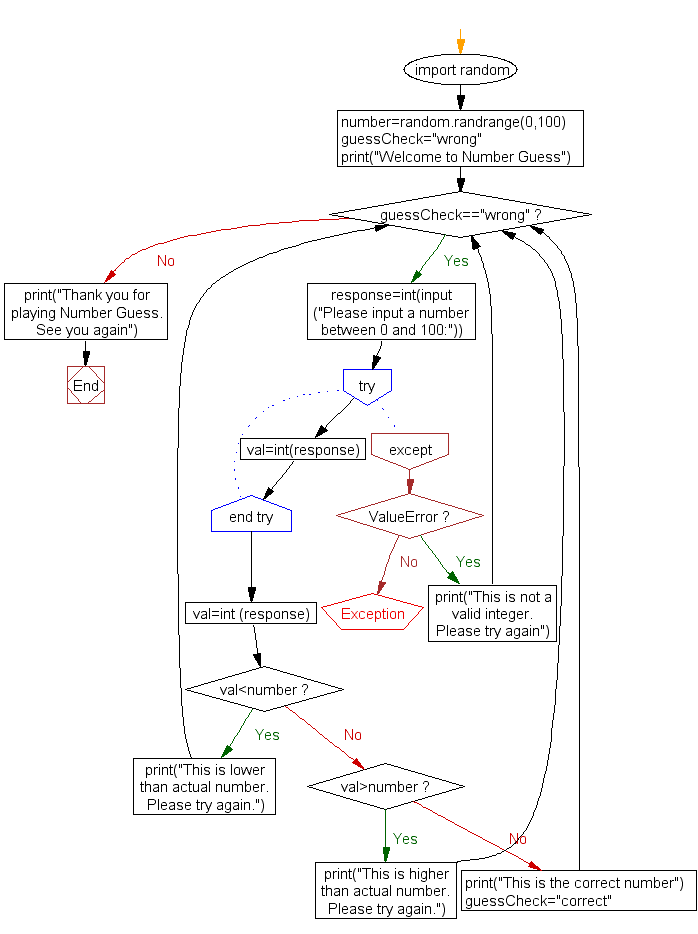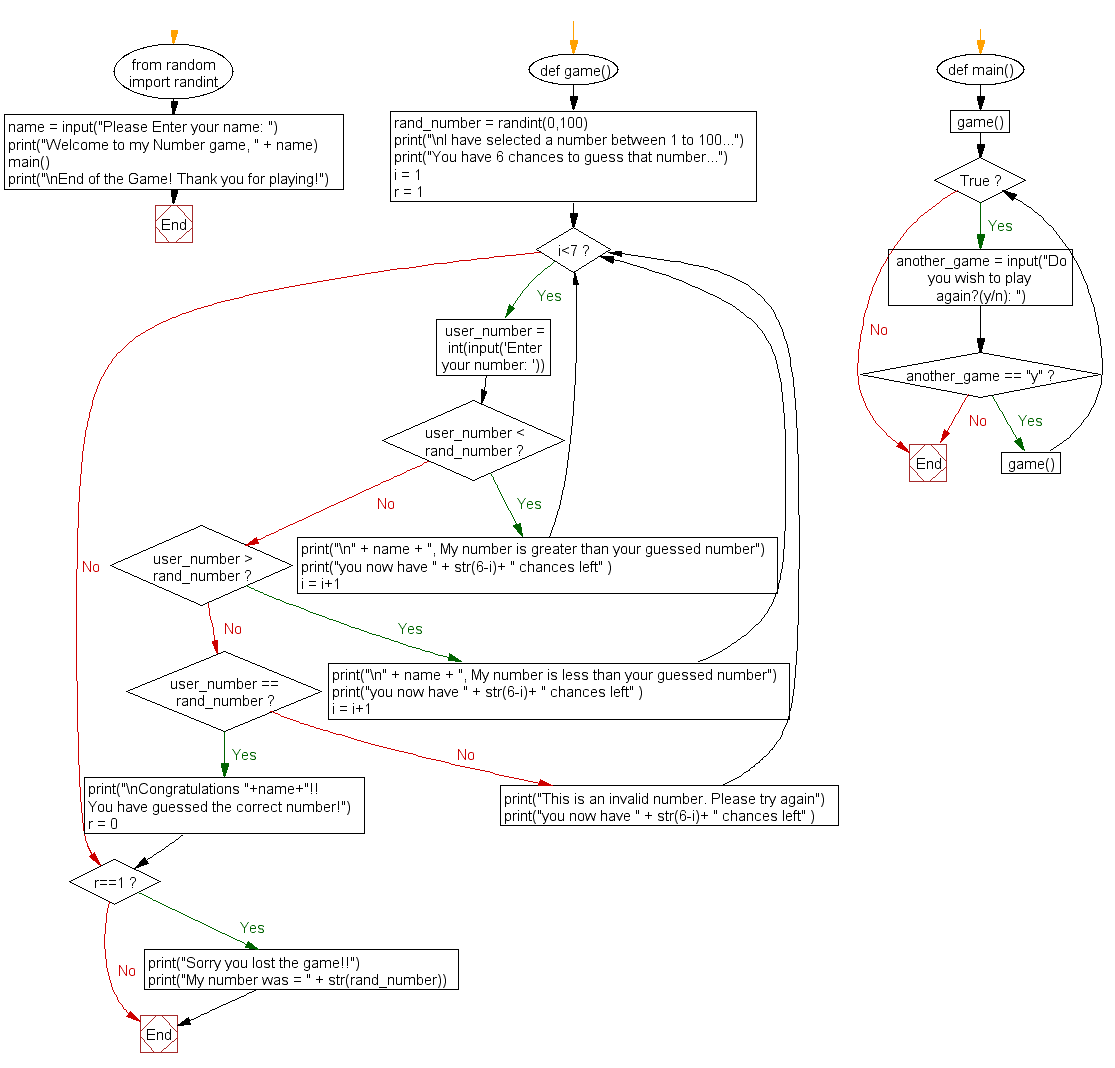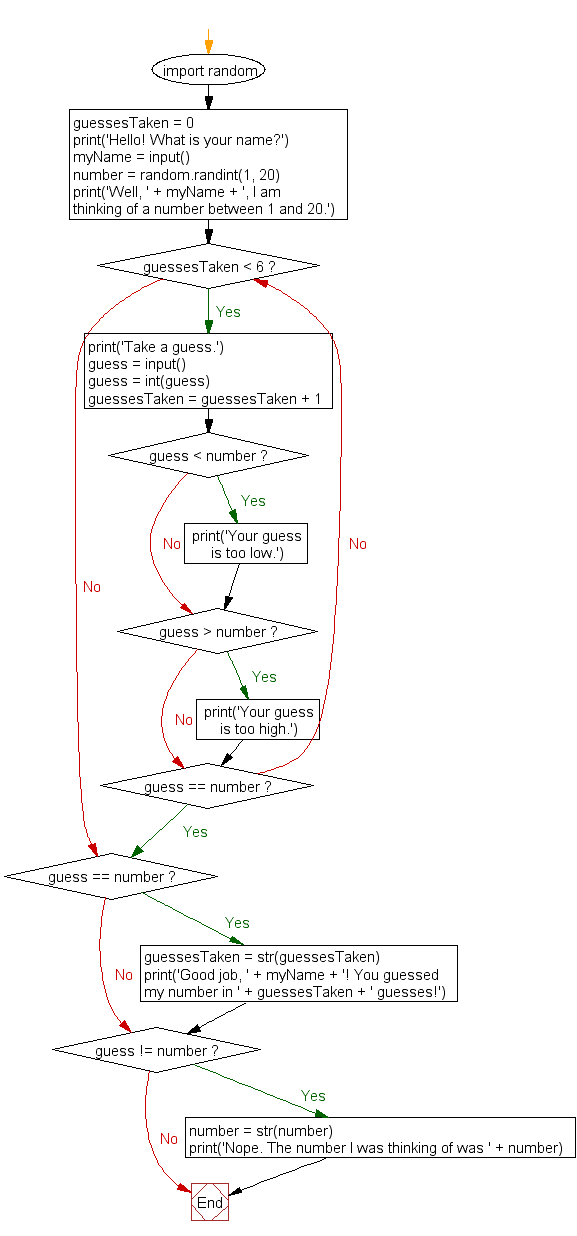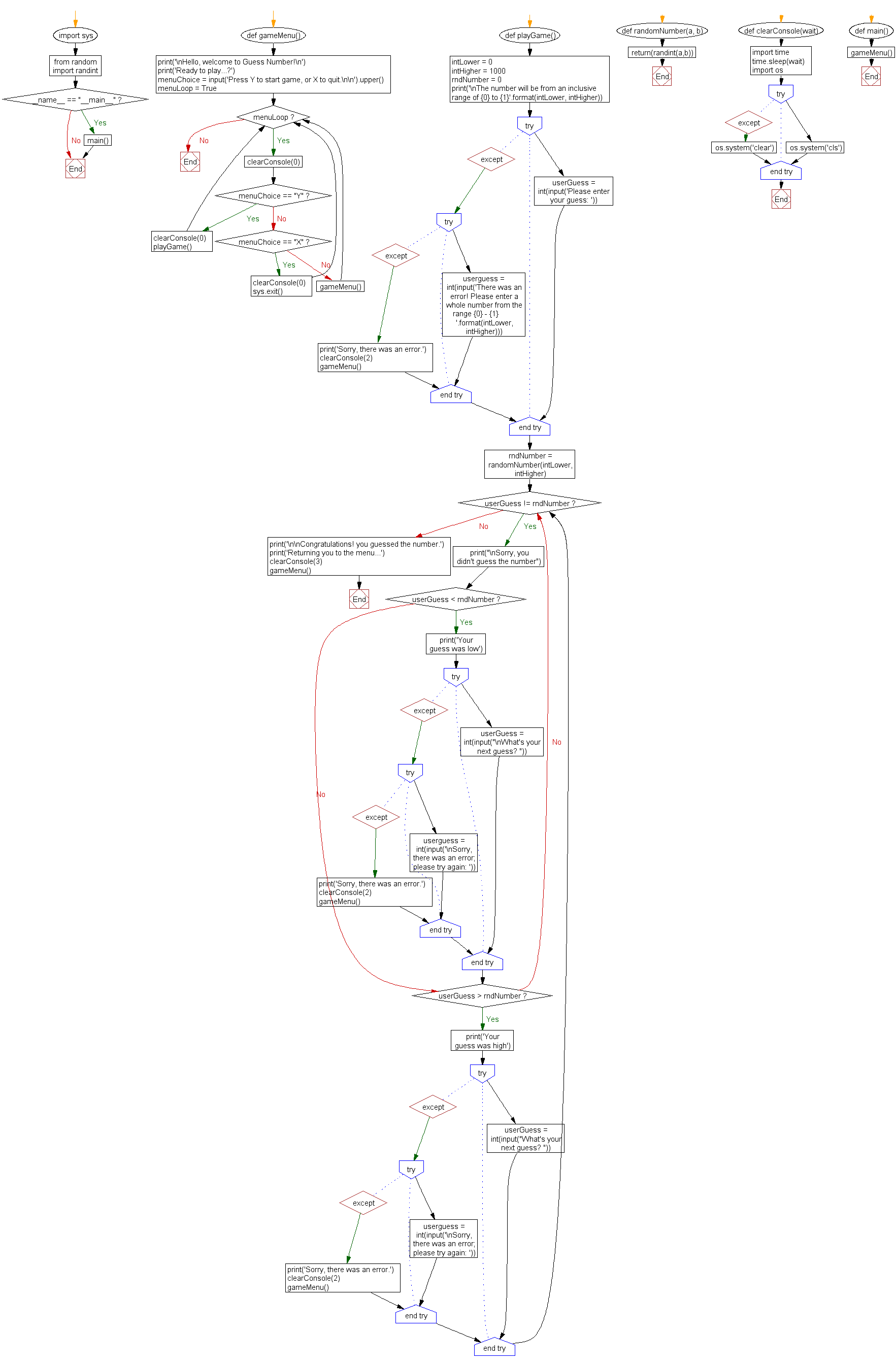﻿ Python Projects: Guess a number that has randomly selected - w3resource

# Python Projects: Guess a number that has randomly selected

## Python Project-3 with Solution

Create a Python project to guess a number that has randomly selected.

Sample Solution -1 :

Python Code:

``````#https://github.com/sarbajoy/NumberGuess/blob/master/numberguess.py
import random
number=random.randrange(0,100)
guessCheck="wrong"
print("Welcome to Number Guess")

while guessCheck=="wrong":
response=int(input("Please input a number between 0 and 100:"))
try:
val=int(response)
except ValueError:
print("This is not a valid integer. Please try again")
continue
val=int (response)
if val<number:
print("This is lower than actual number. Please try again.")
elif val>number:
print("This is higher than actual number. Please try again.")
else:
print("This is the correct number")
guessCheck="correct"

print("Thank you for playing Number Guess. See you again")
```
```

Sample Output:

```Welcome to Number Guess
Please input a number between 0 and 100:6
This is lower than actual number. Please try again.
Please input a number between 0 and 100:25
This is higher than actual number. Please try again.
Please input a number between 0 and 100:15
This is higher than actual number. Please try again.
Please input a number between 0 and 100:10
This is lower than actual number. Please try again.
Please input a number between 0 and 100:11
This is lower than actual number. Please try again.
Please input a number between 0 and 100:123
This is higher than actual number. Please try again.
Please input a number between 0 and 100:12
This is the correct number
Thank you for playing Number Guess. See you again
```

Flowchart:Sample Solution -2 :

You get 6 chances to guess the number that computer has randomly selected from 1 to 100.

Python Code:

``````# Guess a number game
#https://github.com/hardikvasa/guess-the-number-game
#Add feature to check if the input number was between 1-100
#Add levels to the game to make it more exciting

from random import randint  #To generate a random number
print("Welcome to my Number game, " + name)
def game():
rand_number = randint(0,100)   #Generates a random number
print("\nI have selected a number between 1 to 100...")
print("You have 6 chances to guess that number...")
i = 1
r = 1
while i<7:  #6 Chances to the user
user_number = int(input('Enter your number: '))
if user_number < rand_number:
print("\n" + name + ", My number is greater than your guessed number")
print("you now have " + str(6-i)+ " chances left" )
i = i+1
elif user_number > rand_number:
print("\n" + name + ", My number is less than your guessed number")
print("you now have " + str(6-i)+ " chances left" )
i = i+1
elif user_number == rand_number:
print("\nCongratulations "+name+"!! You have guessed the correct number!")
r = 0;
break
else:
print("This is an invalid number. Please try again")
print("you now have " + str(6-i)+ " chances left" )
continue
if r==1:
print("Sorry you lost the game!!")
print("My number was = " + str(rand_number))

def main():
game()
while True:
another_game = input("Do you wish to play again?(y/n): ")
if another_game == "y":
game()
else:
break
main()
print("\nEnd of the Game! Thank you for playing!")
``````

Sample Output:

```Please Enter your name: Jhon Mick
Welcome to my Number game, Jhon Mick

I have selected a number between 1 to 100...
You have 6 chances to guess that number...

Jhon Mick, My number is greater than your guessed number
you now have 5 chances left

Jhon Mick, My number is greater than your guessed number
you now have 4 chances left

Jhon Mick, My number is greater than your guessed number
you now have 3 chances left

Jhon Mick, My number is greater than your guessed number
you now have 2 chances left

Jhon Mick, My number is greater than your guessed number
you now have 1 chances left

Jhon Mick, My number is greater than your guessed number
you now have 0 chances left
Sorry you lost the game!!
My number was = 78
Do you wish to play again?(y/n):
```

Flowchart:Sample Solution -3 :

Python Code:

``````
# This is a guess the number game.
#https://github.com/InterfaithCoding/GuessNumber/blob/master/guess.py
import random

guessesTaken = 0

myName = input()

number = random.randint(1, 20)
print('Well, ' + myName + ', I am thinking of a number between 1 and 20.')

while guessesTaken < 6:
print('Take a guess.') # There are four spaces in front of print.
guess = input()
guess = int(guess)

guessesTaken = guessesTaken + 1

if guess < number:
print('Your guess is too low.') # There are eight spaces in front of print.

if guess > number:

if guess == number:
break

if guess == number:
guessesTaken = str(guessesTaken)
print('Good job, ' + myName + '! You guessed my number in ' + guessesTaken + ' guesses!')

if guess != number:
number = str(number)
print('Nope. The number I was thinking of was ' + number)
``````

Sample Output:

```Hello! What is your name?
Jhon Mick
Well, Jhon Mick, I am thinking of a number between 1 and 20.
Take a guess.
15
Take a guess.
12
Take a guess.
19
Take a guess.
16
Take a guess.
18
Take a guess.
17
Good job, Jhon Mick! You guessed my number in 6 guesses!
```

Flowchart:Sample Solution -4 :

Python Code:

``````#https://github.com/SomeStrHere/GuessNumber/blob/master/GuessNumber.py
import sys
from random import randint

"""Display welcome message and initial menu to user."""

print('\nHello, welcome to Guess Number!\n')
menuChoice = input('Press Y to start game, or X to quit.\n\n').upper()

clearConsole(0)

clearConsole(0)
playGame()

clearConsole(0)
sys.exit()

else :

def playGame() :
"""Obtain input from user, check against random number and display output"""

intLower = 0 #default set to 0
intHigher = 1000 #default set to 1000
rndNumber = 0

print('\nThe number will be from an inclusive range of {0} to {1}'.format(intLower, intHigher))

try :
except :

try :
userguess = int(input('There was an error! Please enter a whole number from the range {0} - {1}\
'.format(intLower, intHigher)))
except :
print('Sorry, there was an error.')
clearConsole(2)

rndNumber = randomNumber(intLower, intHigher)

while userGuess != rndNumber :
print("\nSorry, you didn't guess the number")

if userGuess < rndNumber :

try :
userGuess = int(input("\nWhat's your next guess? "))
except :
try :
userguess = int(input('\nSorry, there was an error; please try again: '))
except :
print('Sorry, there was an error.')
clearConsole(2)

if userGuess > rndNumber :

try :
userGuess = int(input("What's your next guess? "))
except :
try :
userguess = int(input('\nSorry, there was an error; please try again: '))
except :
print('Sorry, there was an error.')
clearConsole(2)

print('\n\nCongratulations! you guessed the number.')
clearConsole(3)

def randomNumber(a, b) :
"""Generates a random int from range a, b"""

return(randint(a,b))

def clearConsole(wait) : #function to clear console on Linux or Windows
"""Clears console, with optional time delay.
Will attempt to clear the console for Windows, should that fail it will attempt to clear the
console for Linux.
"""

import time
time.sleep(wait)
# produces a delay based on the argument given to clearConsole()

import os

try :
os.system('cls') #clears console on Windows

except :
os.system('clear') #clears console on Linux

def main() :

if __name__ == "__main__" :
main()
``````

Sample Output:

```Hello, welcome to Guess Number!

Press Y to start game, or X to quit.

y

The number will be from an inclusive range of 0 to 1000

Sorry, you didn't guess the number

Sorry, you didn't guess the number

Sorry, you didn't guess the number

Sorry, you didn't guess the number

Sorry, you didn't guess the number

Sorry, you didn't guess the number

Sorry, you didn't guess the number

Sorry, you didn't guess the number

Congratulations! you guessed the number.

Hello, welcome to Guess Number!
```

Flowchart:Improve this sample solutions and post your code through Disqus

﻿